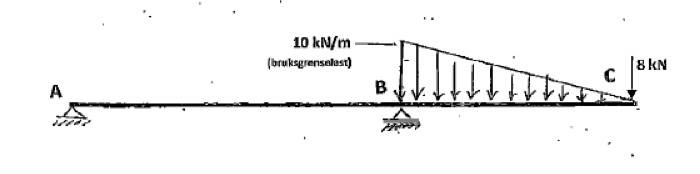# How do I find deflection in this beam?

• raymanmusic

#### raymanmusic

How do I find deflection (displacement) in point C at the end of the beam? Answer is to be given in mm. There is a roller connection (only Fy) in B and an external pin in A (Fy and Fx).As in all beam problems, first determine the reactions at A and B which put the loaded beam in equilibrium.

Once that is done, you can determine the deflection at C from first principles (if you like doing the math) or you can use software which analyzes beam problems.

To find the true deflection of the beam, you will need to know the modulus of elasticity (E) and the moment of inertia (I) of the beam.

I have the modulus of elasticity (E) and the moment of intertia (I), but what formula do I use? There are different formulas for different situations. You can see formulas here: http://en.wikipedia.org/wiki/Deflection_(engineering)

See this attachment: